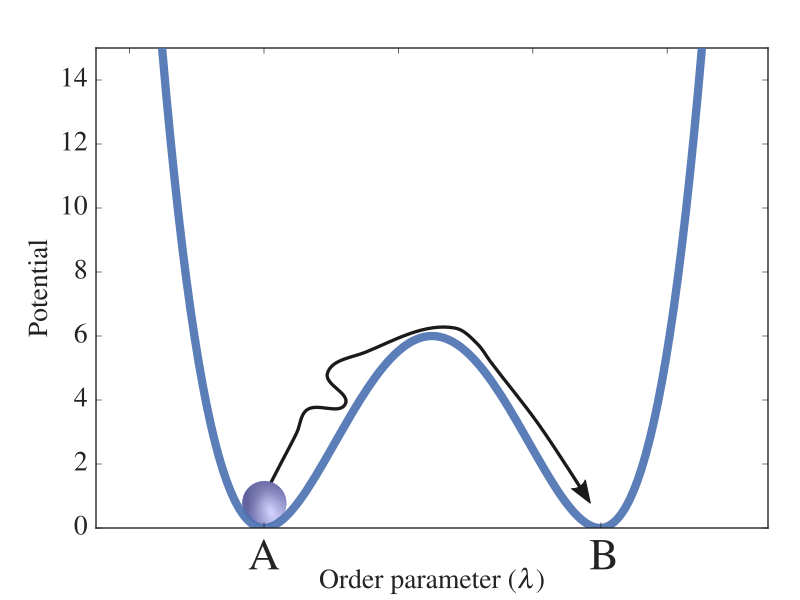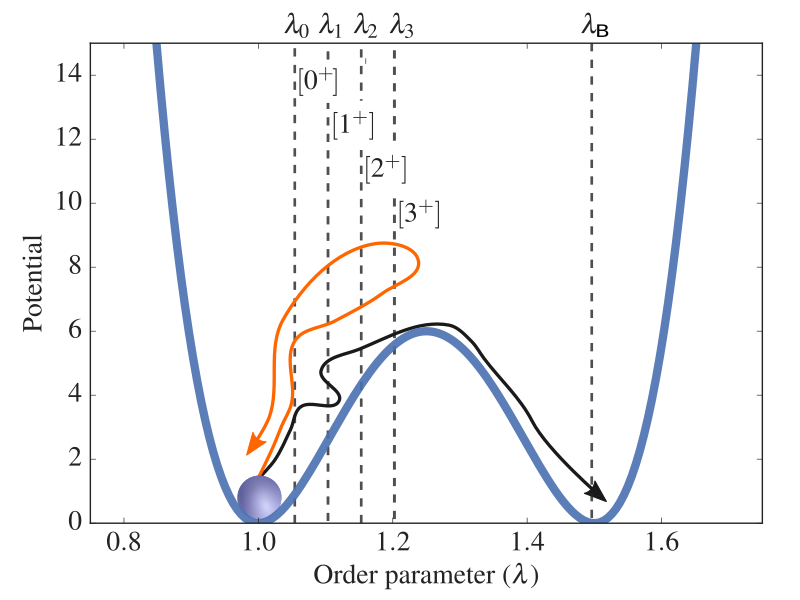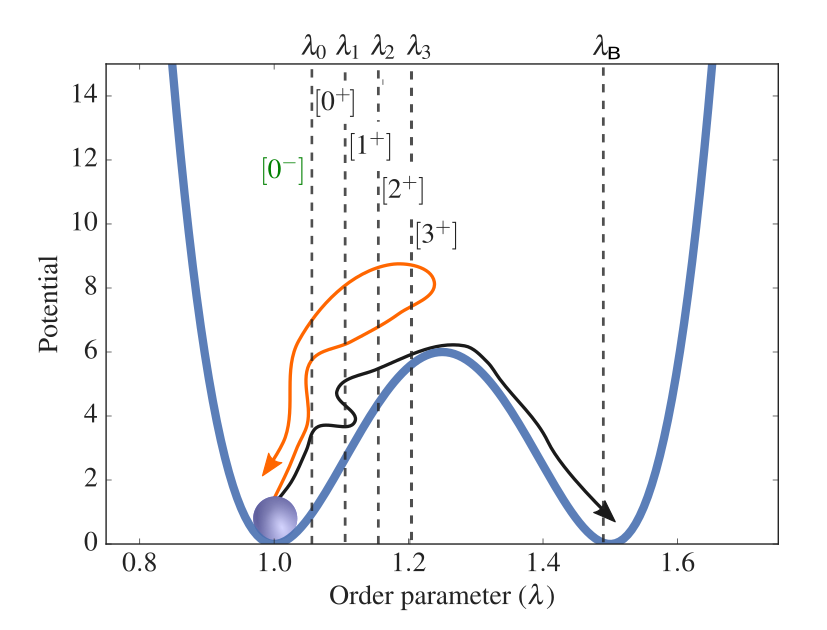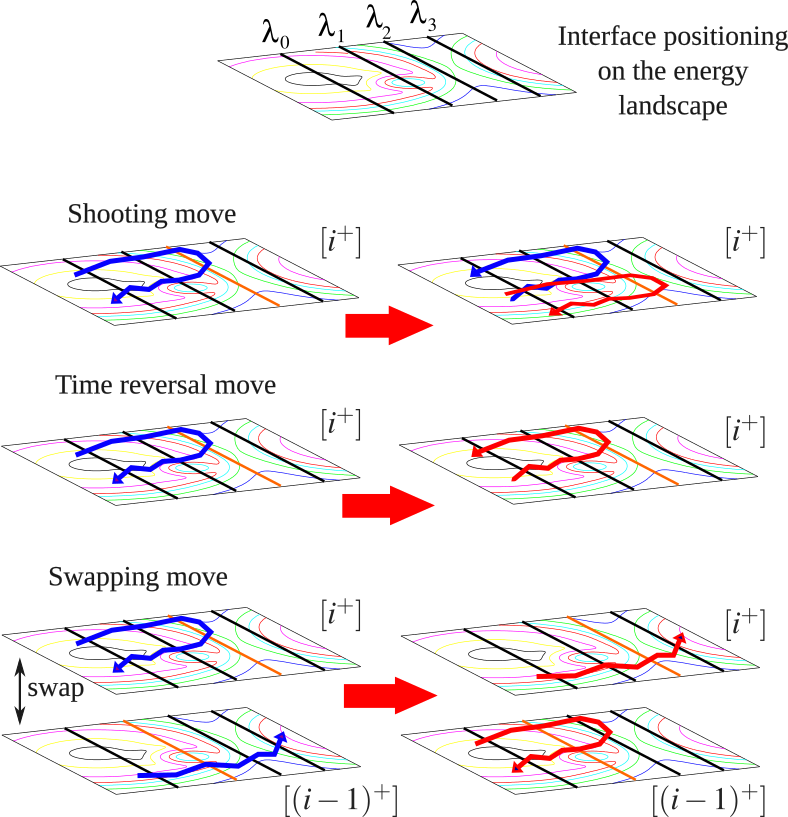# Introduction to PyRETIS and rare event methods¶

PyRETIS is a computational library for performing molecular simulations of rare events with a focus on transition interface sampling (TIS)  and replica exchange transition interface sampling (RETIS) .

Rare events are called rare because they happen at time or length scales much longer than we are able to simulate through brute-force simulations. Raindrops form every day, but compared to the motion of water molecules, raindrop formation is rare, and simulating it through brute-force simulations would take forever and a day.

Rare event methods aim to sample these rare events inaccessible through brute-force simulation.

## Transition Interface Sampling¶

To explain the basic concepts, we consider an illustrative example: The transition between two stable states, from the reactant (labeled A) to the product (labeled B) as illustrated in Fig. 1.Fig. 1 A potential energy barrier separating two stable states A and B. The order parameter measures the extent of the reaction in this particular energy landscape and a possible trajectory for a particle making the transition is illustrated as a black arrow.

These two states are defined by a progress coordinate (or order parameter),, where state A is forand state B for. The central quantity calculated in TIS/RETIS simulations is the rate constantfor the transitionwhich can be expressed as:and we see that it contains two parts:

• The initial fluxwhich measures how often trajectories start off at the foot of the reaction barrier from the reaction side. In TIS this is obtained from a molecular dynamics (MD) simulation which in PyRETIS is requested by running a md-flux simulation. Note that this is not needed for the RETIS method as explained.
• The crossing probabilityof reachingbeforegiven thathas just been crossed. In PyRETIS this can be accomplished by running a tis or retis simulation.

As this is a rare event, the crossing probability is extremely small and nearly impossible to compute in brute-force simulations. Transition interface sampling divides the region between A and B into sub-regions using interfaces, denoted(see Fig. 2). The first interface,is positioned at the interface defining state A (), and the next interface,is put atsuch that the probability of reachingfromis no longer extremely small. We can continue in this fashion and placemore interfaces until we reach. What we effectively have done with theseinterfaces is to split up the computation of the extremely small crossing probability into the computation of many, not-so small, crossing probabilities:Here, the intermediate crossing probabilities,, are formally defined as the probability of a path crossinggiven that it originated from, ended inor, and had at least one crossing within the past.

The interfaces we have placed defines the so-called path ensembles. A path ensemble comprises all possible trajectories that start at the foot of reaction barrier from the reactant side (), end in it or at the product region () and having reached a certain threshold value () between the start point and the final point. This path ensemble is labelled as. The probabilities,can then be obtained as the fraction of paths in theensemble that also cross.Fig. 2 Illustration of TIS interfaces placed along the order parameter in a system where a potential energy barrier separates two stable states A and B. The interfaces define different ensembles and here, two trajectories are shown. One (black) is reactive, reaching the final state, while the other (orange) just reaches the intermediateinterface.

What is left now, is to have an efficient way of generating trajectories for the various path ensembles. This is in fact done by making use of a selection of Monte Carlo (MC) moves. For TIS  we choose between two moves:

• The shooting move which is adapted from the transition path sampling (TPS) shooting algorithm   to allow variable trajectory length. In this move, we generate a new trajectory from an existing trajectory one by:

1. Picking randomly one of the discrete MD steps in the present trajectory.
2. Modifying the velocities of this phase point (e.g. by randomly drawing new velocities from a Maxwellian distribution).
3. Generating a new trajectory from this new phase point by integrating (i.e. running MD simulations) forward and backward in time until A or B is reached. The new trajectory is then obtained by merging the backward and forward trajectories.

The new trajectory is accepted as part ofonly if all the following criteria are satisfied:

1. A detailed balance condition for the energy and path length. 
2. It starts at3. It has at least one crossing withbefore ending in A or B.

The shooting move gives a much higher chance to generate a valid trajectory at each trial compared to simply starting from a random phase point within the reactant well.

• The time reversal move which generates trajectories by simply changing the time direction of a path. 

These two moves are illustrated in Fig. 4.

## Replica Exchange Transition Interface Sampling¶

The RETIS method is similar to TIS and employs both the shooting and time reversal moves. In addition, RETIS makes use of the swapping move and defines a new ensemblewhich consist of trajectories that explore the reactant state (see the illustration in Fig. 3).Fig. 3 Illustration of RETIS interfaces placed along the order parameter in a system where a potential energy barrier separates two stable states A and B. The interfaces define different ensembles and here, two trajectories are shown. One (black) is reactive, reaching the final state, while the other (orange) just reaches the intermediateinterface. In RETIS a special path ensemble,is also considered as described in the text.

The swapping move acts between different path simulations. If two simulations generate simultaneously two paths that are valid for each other’s path ensemble, these two paths can be swapped. The swapping moves increase with negligible extra computational cost the number of accepted paths in the ensembles and decrease significantly the correlations between the consecutive paths within the same ensemble. All the moves used for generating trajectories are illustrated in Fig. 4.Fig. 4 Illustration of the RETIS moves for generating trajectories. A contour plot of a hypothetical free energy surface along a progress coordinate and an arbitrary second coordinate is shown and 4 interfaces (,,and) have been positioned along the progress coordinate. Three different RETIS moves (shooting, time reversal and swapping) are shown for thepath ensemble. The old paths are in blue and the new paths after (a successful) completion of the MC moves are shown in red. The orange line show the interface that needs to be crossed for a valid path in the current ensemble.

There is one notable exception where the swapping move is more computationally demanding: the swap between theandensembles requires MD simulations. 

In a TIS simulation, as explained above, we have to perform an extra simulation in order to calculate the initial flux. In RETIS, this initial flux can directly be obtained by:whereis the average path length in theensemble andthe average path length in theensemble.

In PyRETIS, RETIS simulations are requested by setting the simulation task to RETIS.